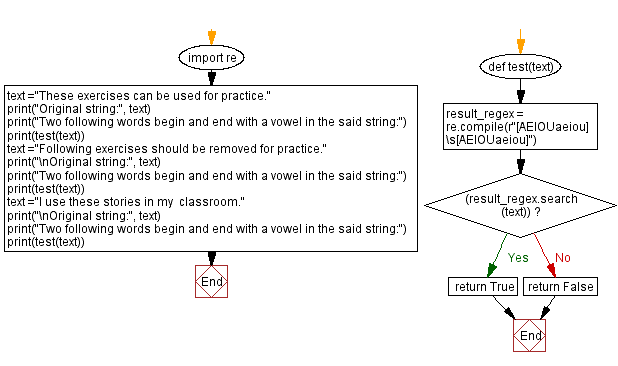﻿ Python - Two following words begin and end with a vowel# Python Exercises: Two following words begin and end with a vowel

## Python Regular Expression: Exercise-58 with Solution

From Wikipedia,
A vowel is a syllabic speech sound pronounced without any stricture in the vocal tract. Vowels are one of the two principal classes of speech sounds, the other being the consonant.

Write a Python program that takes a string with some words. For two consecutive words in the said string, check whether the first word ends with a vowel and the next word begins with a vowel. If the program satisfy the said condition, return true other false. Only one space is allowed between the words.

Sample Data:
("These exercises can be used for practice.") -> True ("Following exercises should be removed for practice.") -> False ("I use these stories in my classroom.") -> True

Sample Solution-1:

Python Code:

``````import re
def test(text):
return bool(re.findall('[AEIOUaeiou] [AEIOUaeiou]', text))

text ="These exercises can be used for practice."
print("Original string:", text)
print("Two following words begin and end with a vowel in the said string:")
print(test(text))
text ="Following exercises should be removed for practice."
print("\nOriginal string:", text)
print("Two following words begin and end with a vowel in the said string:")
print(test(text))
text ="I use these stories in my  classroom."
print("\nOriginal string:", text)
print("Two following words begin and end with a vowel in the said string:")
print(test(text))
```
```

Sample Output:

```Original string: These exercises can be used for practice.
Two following words begin and end with a vowel in the said string:
True

Original string: Following exercises should be removed for practice.
Two following words begin and end with a vowel in the said string:
False

Original string: I use these stories in my  classroom.
Two following words begin and end with a vowel in the said string:
True
```

Flowchart:Sample Solution-2:

Python Code:

``````import re
def test(text):
result_regex = re.compile(r"[AEIOUaeiou]\s[AEIOUaeiou]")
if (result_regex.search(text)):
return True
return False

text ="These exercises can be used for practice."
print("Original string:", text)
print("Two following words begin and end with a vowel in the said string:")
print(test(text))
text ="Following exercises should be removed for practice."
print("\nOriginal string:", text)
print("Two following words begin and end with a vowel in the said string:")
print(test(text))
text ="I use these stories in my  classroom."
print("\nOriginal string:", text)
print("Two following words begin and end with a vowel in the said string:")
print(test(text))
```
```

Sample Output:

```Original string: These exercises can be used for practice.
Two following words begin and end with a vowel in the said string:
True

Original string: Following exercises should be removed for practice.
Two following words begin and end with a vowel in the said string:
False

Original string: I use these stories in my  classroom.
Two following words begin and end with a vowel in the said string:
True
```

Flowchart:Python Code Editor:

Have another way to solve this solution? Contribute your code (and comments) through Disqus.

Previous Python Exercise: Convert a given string to snake case.
Next: Python NumPy Array Object Exercises Home.

What is the difficulty level of this exercise?

Test your Programming skills with w3resource's quiz.

﻿

## Python: Tips of the Day

Getting sorted iterable (can sort by "compare" function):

```>>> a = [1, 2, -3]
>>> sorted(a)
[-3, 1, 2]

>>> sorted(a,key=abs)
[1, 2, -3]
```